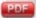RIMARC Implementation

## RIMARC

### Ranking Instances by Maximizing the Area under ROC Curve

Java implementation: RIMARC.jar

Publication:

H. Altay Güvenir, Murat Kurtcephe, Ranking Instances by Maximizing the Area under ROC Curve, IEEE Transactions on Knowledge and Data Engineering,Vol. 25, No. 10, (2013), pp 2356-2366.

Execution samples:

```\$ java -jar RIMARC.jar
RIMARC dataFile [-x n] [-t n] [-v n]
The dataFile is the name of the file containing the data, in tab separeted values format.
If -x n is given:
Applies and Returns n fold cross-validation results.
If -t n is given:
The first n instances in the dataset is used as the test set, and the remaining as train set.
If -v n is given:
Verbosity level is set to n. Possible values are 0,1,2,3 or 4. Default: 0
If -x or -t is not given the dataset is used as both train and test sets.
```
Here, `dataFile` is the name of the file that contains the dataset. It must be in tab separated values format. The first line of the file contains the names of the features. The first column contains the `class` (dependent) feature. The label `P` represents the positive class, and the label `N` represents the negative class.

The dataFile sampleDataSet.txt contains three features; namely, `categoricF`, `ordinalF` and `numericalF`. It may be obtained by saving as "Tab delimited" from the sampleDataSet.xlsx.

Example runs:

1. `\$> java -jar RIMARC.jar sampleDataSet.txt`

The same dataset is used for both training and test. The output is

```AUC= 1.0 Training time= 3 ms. Testing time= 0 ms.
Complete run time: 16 ms.
```
The rule file `sampleDataSet.txt_rules.txt` is generated.

2. `\$> java -jar RIMARC.jar sampleDataSet.txt -v 1`

The same dataset is used for both training and test. The output is

```AUC= 1.0 Training time= 3 ms. Testing time= 0 ms.
Complete run time: 16 ms.
```
The rule file and the log file `sampleDataSet.txt.log` are produced.

3. `\$> java -jar RIMARC.jar sampleDataSet.txt -t 2 -v 3`

The first 2 instances of the dataset are used for testing, the remaining instances are used to learn the ranking model. The output is

```AUC= 1.0 Training time= 3 ms. Testing time= 0 ms.
Complete run time: 22 ms.
```
The rule file and the log file `sampleDataSet.txt-t2.log` are produced.

4. `\$> java -jar RIMARC.jar sampleDataSet.txt -x 2 -v 3`

2-fold stratified cross-validation. The output is

```Overall AUC= 0.95, Average Training time: 2 ms., Average Testing time: 1 ms.
Complete run time: 14 ms.
```
The rule file, the log file `sampleDataSet.txt-x2.log`, and the score file `sampleDataSet.txt_score.txt` are produced. The score file lists the class labels and the computed score of the instances. The `ID` column represents the row number of the instance in the dataFile; note that, due to the stratification, the order of the instances may be different than their order in the dataFile.